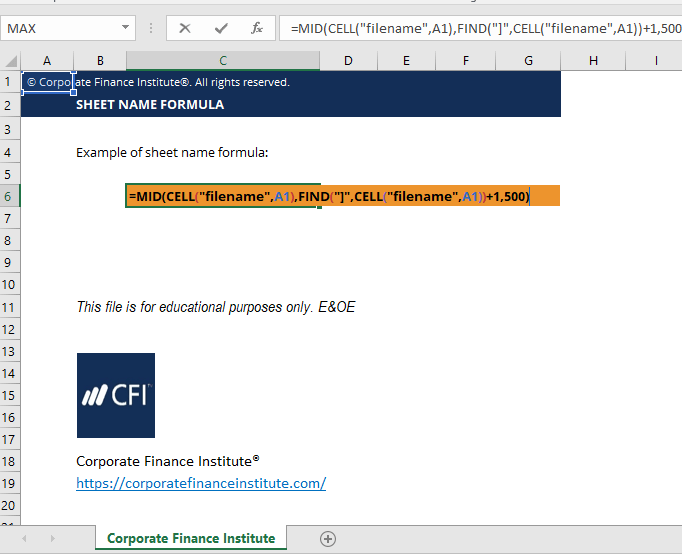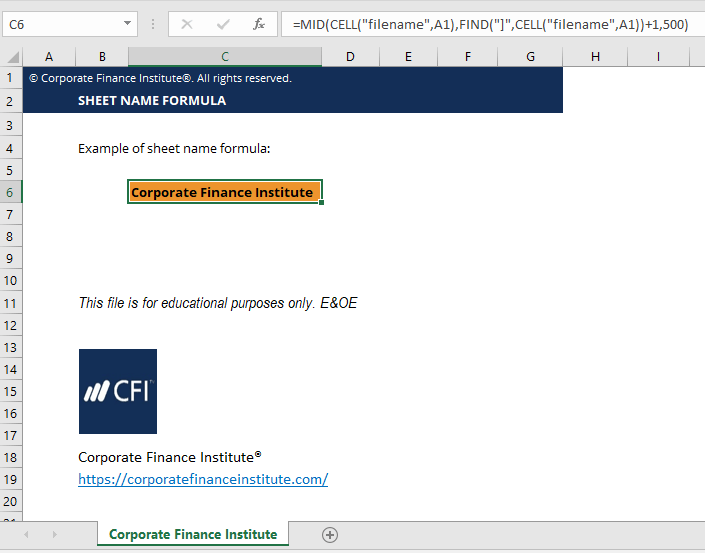# Sheet Name Code

This article outlines how to display the name of a sheet in Excel.

## What is sheet name code Excel formula?

In Excel, if you want to display the name of a Sheet in a cell, you can use a combination of formulas to display it. Using the sheet name code Excel formula requires combining the MID, CELL, and FIND functions into one formula.

For example, if you are printing out a financial model onto paper or as a PDF, then you may want to display the sheet name on the top of each page.  You can now easily do so by using this formula.

### Sheet name code Excel formula

=MID(CELL(“filename”,A1),FIND(“]”,CELL(“filename”,A1))+1,500)

(See screenshots below)

Example of creating the sheet name code Excel

Step 1: Type “CELL(“filename”,A1)”. The cell function is used to get the full filename and path. This function returns the filename of .xls workbook, including the sheet name.  This is our starting point, and then we need to remove the file name part and leave only the sheet name.

Step 2: Type “FIND(“]”,CELL(“filename”)+1,500″.  Essentially what the formula does is go into the file name and find the “]” which is where the workbook name ends.  From there it moves over one character (+1) to where the sheet name starts.  Finally, we add 500 to the end, or any number that is larger than the number of characters a sheet name would realistically have.

Congratulations, you have now displayed the name of a sheet in Excel!

### Sheet name code Excel template### More excel development

This has been CFI’s guide to the sheet name code Excel formula. To keep learning and expanding your skill set and knowledge base as a financial analyst, we highly recommend these additional resources to help you advance your career.  Some of our most popular resources from CFI include:

• Excel for dummies
• Index and Match functions
• Excel shortcuts list
• Important Excel formulas
• Free online courses

### Free Excel Tutorial

To master the art of Excel, check out CFI’s FREE Excel Crash Course, which teaches you how to become an Excel power user.  Learn the most important formulas, functions, and shortcuts to become confident in your financial analysis.

Launch CFI’s Free Excel Course now to take your career to the next level and move up the ladder!